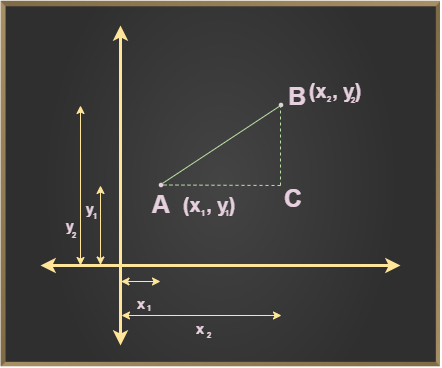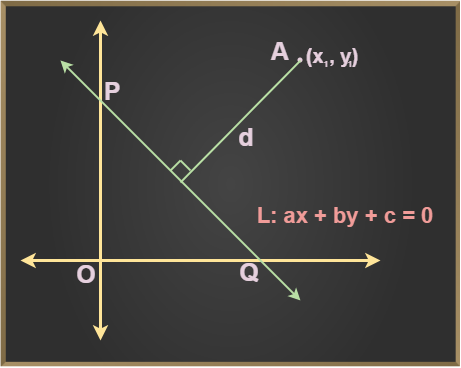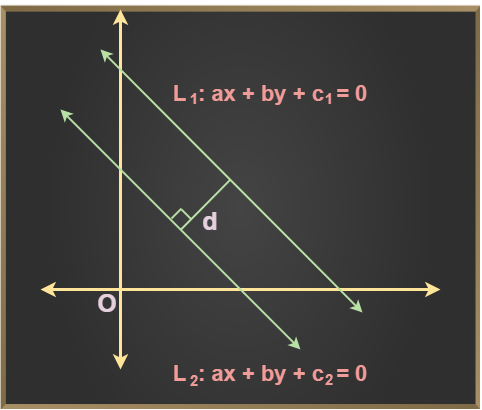Open in App
Not now

# Distance Formula

• Last Updated : 09 Dec, 2022

Distance formula is used to compute the distance between two points, a point, and a line, and two line segments. The distance formula can be used for the computation of the sum of the lengths of all the sides of a polygon, the perimeter of polygons on a coordinate geometry, the area of polygons, etc. The lengths of the sides of triangles can also be computed using the distance formula. The type of triangle, scalene, isosceles or equilateral can also be determined using the distance formula.

## What is Distance Formula?

The x coordinate of the point is known as the abscissa whereas the y coordinate is known as the ordinate. The distance formula can be used to calculate the distance between two points of the XY-plane. An ordered pair (x, y) represents the coordinate of the point, where the x-coordinate is defined as the distance of the point from the x-axis and the y-coordinate is the distance of the point from the y-axis.

## Distance Between Two Points

The formula used to find the distance between two points in a 2-d plane is given by,

Consider any two arbitrary points A (x1, y1) and B (x2, y2) on the given coordinate axis. The distance between the following two points is given as follows:d = √{ (x2 – x1)2 + (y2 – y1)2 }

## Derivation of the Distance Formula

Derivation of the Distance Formula is given using the Pythagoras theorem. In the right-angled triangle ABC, we have,

AB2 = AC2 + BC2

Distance between points A and C is (x2 – x1)

Distance between points B and C is (y2 – y1)

Distance, d is calculated as,

d2 = (x2 – x1)2 + (y2 – y1)2

Now,

Taking the square root on both sides,

d = √[(x2 – x1)2 + (y2 – y1)2]

Thus, d is the distance between two points

## Distance Formula Between a Point and a Line

The distance between a point and a line is given by the formula, let’s take a point (x1, y1) and a line ax + by + c = 0d =## Distance Formula Between Two Parallel Lines

The distance between two parallel lines is given by the formula, let’s take two parallel line ax + by + c1 = 0 and ax + by + c2 = 0d =## Solved Examples on Distance Formula

Example 1: Calculate the distance between the points X(5, 15) and Y(4, 14)

Solution:

Distance between point X and Y is given by distance formula

d = √[( x2 – x1 )2 + ( y2 – y1 )2]

d = √[( 4 – 5 )2 + ( 14 – 15 )2]

d = √[( 1 )2 + ( 1 )2]

d = √(2)

Distance between X and Y is √2 or 1.41

Example 2: Find the distance between the parallel lines -6x + 20y + 10 = 0 and -6x + 20y + 20 = 0.

Solution:

General equation of parallel lines is

Ax + By + C1 = 0 and Ax + By + C2 = 0,

Here,

A = -6,
B = 20,
C1 = 10,
C2 = 20

Applying formula

d =d =d = 10 / √436

Thus, the distance between two parallel lines is d = 10 / √436

Example 3: Calculate the distance between line 4a + 6b – 26 = 0 from the point (2, –4).

Solution:

General equation of parallel lines is

A point (x1, y1) and a line ax + by + c = 0

Here,

A = 4, B = 6 and C = –26

Applying formula

d =d =d = -42 / √52

Thus, the distance between lines and point is d = -42 / √52

Example 4: Calculate the distance between points A(-25, -5) and B(-16, -4).

Solution:

Distance between point A and B is given by distance formula

d = √[( x2 – x1 )2 + ( y2 – y1 )2]

d = √[( (-16) – (-25) )2 + ( (-4) – (-5) )2]

d = √[( 9 )2 + ( 1 )2]

d = √(82)

Distance between A and B is √82 or 9.05

## FAQs on Distance Formula

Question 1: How is Pythagorean Theorem related to the Distance Formula?

The distance between two points (x1,y1) and (x2,y2) is calculated by,

Distance2 = (x2−x1)2+(y2−y1)2

Distance = √(x2−x1)2+(y2−y1)2

where, (x1,y1)and (x2,y2) are two vertices of a right-angled triangle that form its hypotenuse when joined.

Question 2: What is Manhattan Distance Formula?

The Manhattan Distance is the distance measured between two points along the right angular axis. Manhattan Distance between two points (x1, y1) and (x2, y2) is given by the formula | x2 – x1 | +  | y2 – y1 |

Question 3: What is the coordinate of a point?

The ordered pair (x,y) which is used to know the position of any point in the Cartesian plane is called the coordinate point. For example, (1,3) is a coordinate point.

Question 4: What is Distance Formula?